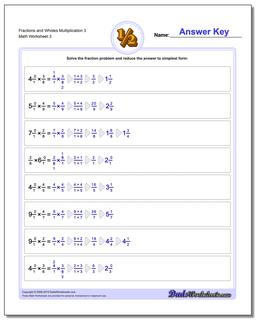# Math Worksheets: Fraction Multiplication: Fraction Multiplication: Fractions and Wholes Multiplication 3 (Third Worksheet)## Fractions and Wholes Multiplication 3 (Third Worksheet)

PropertyValue
DescriptionFractions and Wholes Multiplication 3: Basic multiplying fractions against wholes. Each problem on these worksheets has a single fraction multiplied by a whole value. (Third Worksheet)
Resource TypeWorksheet